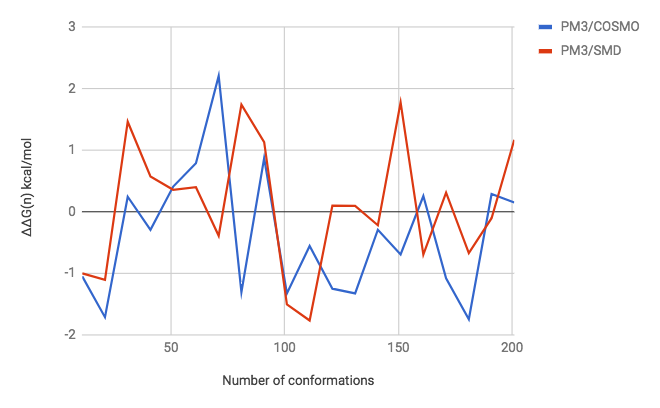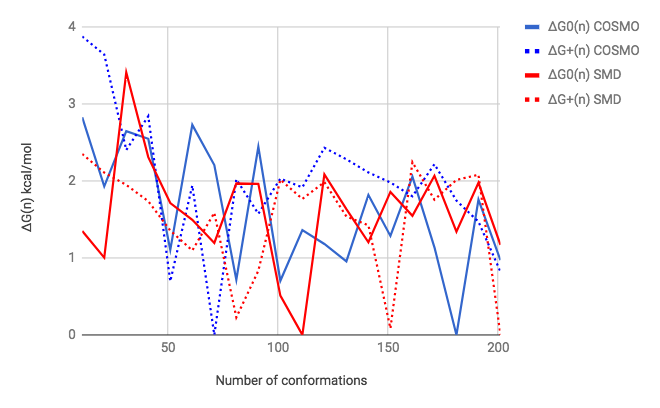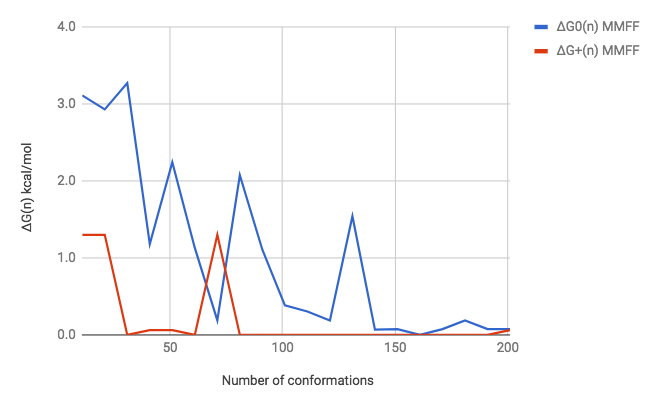## Thursday, December 28, 2017

### Predicting pKa values using PM3 - conformer search part 2Disclaimer: These are preliminary results and may contain errors
In a previous post a looked at the effect of conformational search on the accuracy of pKa predictions.  This is a follow up from a slightly different angle using data obtained by Mads.

The plot shows
$$\Delta \Delta G(n) = G_{0}(n) - G_{+}(n) - (G_{0}(\min) - G_{+}(\min))$$
where $G_{0}(n)$ is the minimum free energy value found among $n$ conformations of neutral acebutolol generated by RDKit and optimized using either PM3/COSMO (blue) or PM3/SMD (red). $G_{+}(n)$ is the corresponding value for protonated acebutolol.  $G_{0}(n)$ (and $G_{+}(n)$) are found using $n = 11, 21, 31, ..., 201$ starting conformations and $G_{0}(\min)$ is the lowest value of  found for $G_{0}(n)$ and similarly for $G_{+}(\min)$.

So $G_{0}(\min) - G_{+}(\min)$ is our best estimate of the correct $\Delta G$ value and $\Delta \Delta G(n)$ is the deviation from the best estimate for a give value of $n$.

Keeping in mind that a 1.4 kcal/mol error corresponds to a 1 pH unit error in pKa, we are clearly no where near convergence. The fact that we observe this using two different programs (MOPAC and GAMESS) indicates that the problem is probably not the optimizer.The next plot shows $\Delta G_X(n) =G_X(n)-G_X(\min)$. All errors are above 1 kcal/mol for $n<50$. The error for COSMO neutral does not dip below 0.5 kcal/mol until $n =$ 181, compared to $n=$ 101 for SMD neutral.The RDKit starting geometries are not pre-minimized using MMFF. The above plot shows the corresponding plot for MMFF optimized in the gas phase.  I think the much better convergence is due to the optimization being done in the gas phase.  It's interesting that, again, the neutral is slower to converge, and only does so for $n >$ 140.

I think the next step is do redo this analysis with focus on finding the lowest energy conformer rather than the accuracy of the pKa values.